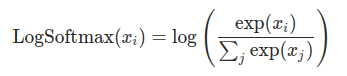# "log_softmax function" in pytorch tutorial example

I am studying the link below.
https://pytorch.org/tutorials/beginner/nn_tutorial.html

But I can’t understand “log_softmax” written in this document.

``````def log_softmax(x):
return x - x.exp().sum(-1).log().unsqueeze(-1)
``````

How this function match to the figure below?Hi Dong Wook!

My guess is that you’re being thrown off by the “log-sum-exp trick”
that is being used to rewrite the “standard” expression for
`log_softmax` in a (mathematically-equivalent) form that avoids
floating-point overflow / underflow problems when evaluating
the expression numerically.

See the “log-sum-exp trick for log-domain calculations” section of
is not specifically about the `log_softmax` function, but instead
about the related LogSumExp function. `log_softmax` has the